# RD Sharma Solutions Class 7 Chapter 19 Visualising Solid Shapes

Read RD Sharma Solutions Class 7 Chapter 19 Visualising Solid Shapes below, students should study RD Sharma class 7 Mathematics available on Studiestoday.com with solved questions and answers. These chapter wise answers for class 7 Mathematics have been prepared by teacher of Grade 7. These RD Sharma class 7 Solutions have been designed as per the latest NCERT syllabus for class 7 and if practiced thoroughly can help you to score good marks in standard 7 Mathematics class tests and examinations

Exercise 19.1

Question :1. Complete the following table and verify Euler’s formula in each case.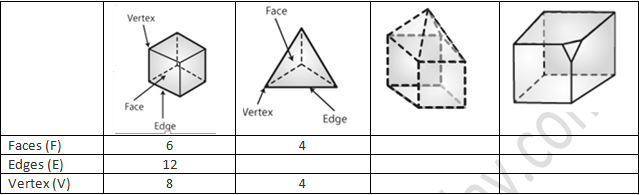Solution 1: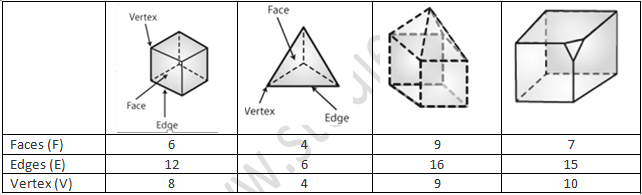According to Euler’s formula is (F – E + V)

(i) (F – E + V)

= (6 – 12 + 8) = 2

So, Euler’s formula is verified

(ii) (F – E + V)

= (4 – E + 4) = 2.

E = 6

So, Euler’s formula is verified

(iii) (F – E + V)

= (9 – 16 + 9) = 2.

So, Euler’s formula is verified

(iv) (F – E + V)

= (7 – 15 + 10) = 2.

So, Euler’s formula is verified

Question :2. Give three examples from our daily life which are in the form of

(i) A cone

(ii) A sphere

(iii) A cuboid

(iv) A cylinder

(v) A pyramid.

Solution 2:

(i) A Cone: Party hat, Ice cream cone, Christmas tree.

(ii) A Sphere: Ball, Planets, Moon.

(iii) A Cuboid: Bricks, Book, Mattresses.

(iv) A Cylinder: Pipes, Cold drink cans, Battery

(v) a Pyramid: Christmas tree, prism, piece of cake

Exercise 19.2

Question :1. Match the following nets with appropriate solids: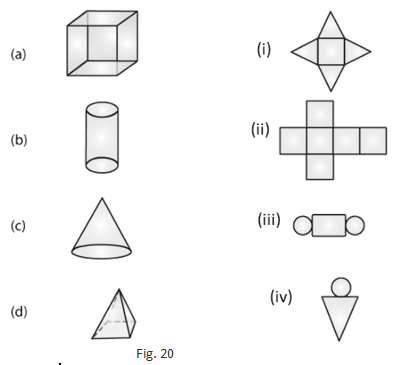Solution 1:Question :2. Identify the nets which can be used to make cubes (cut-out the nets and try it):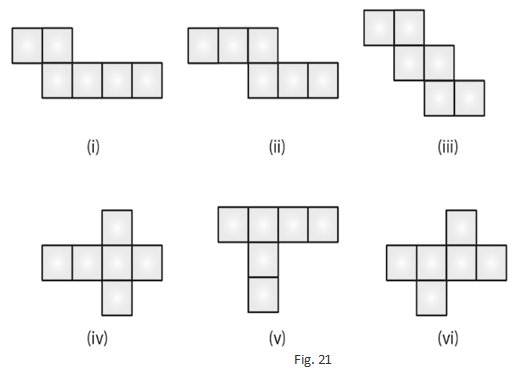Solution  2:

(ii), (iv) and (vi) form a cube.

Question :3. Can the following be a net for a die? Explain your answer.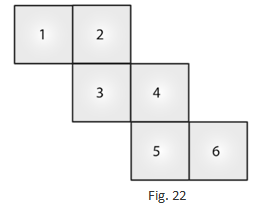Solution 3:

We know that in a die, the sum of the number of opposite faces of a die is 7. In the specified figure, it is not possible to get the sum as 7. As a result, the given net is incompatible with a die.

Question :4. Out of the following four nets there are two correct nets to make a tetrahedron. Identify them.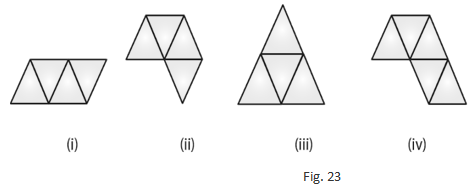Solution 4:

For making a tetrahedron, (i) and (iii) are suitable nets.

Question :5. Here is an incomplete net for making a cube. Complete it in at least two different ways.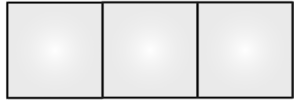Solution 5: